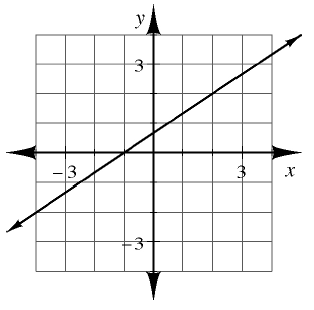### Home > CCA > Chapter Ch4 > Lesson 4.3.1 > Problem4-105

4-105.

Multiple Choice: Martha’s equation has the graph shown at right. Which of these are solutions to Martha’s equation? (Remember that more than one answer may be correct.)1.  $\quad ( - 4 , - 2 )$

1.  $( - 1,0 )$

1.  $x = 0 \text { and } y = 1$

1.  $x = 2 \text { and } y = 2$

Which of the given ordered pairs is on the line?